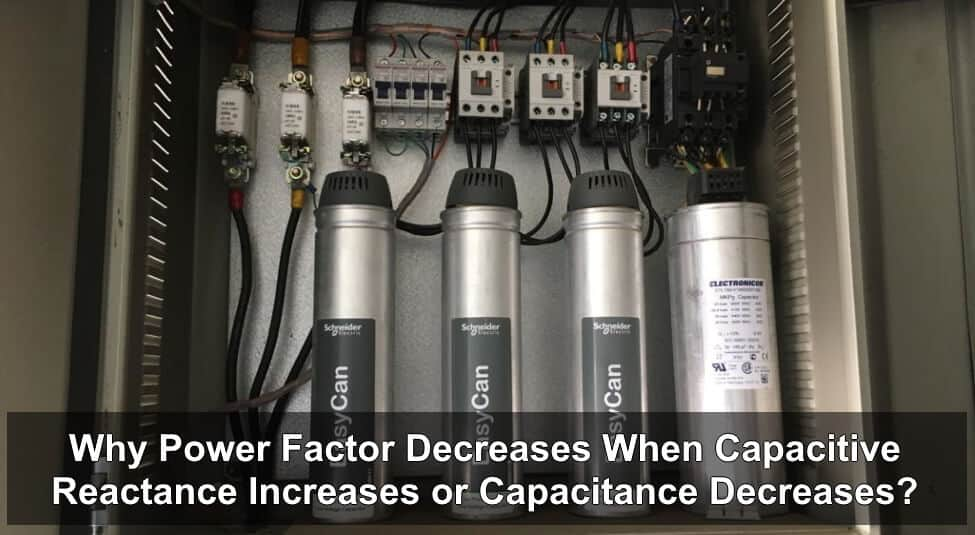# Why Power Factor Decreases When Capacitive Reactance Increases or Capacitance Decreases?

## In a Capacitive Circuit, Why the Power Factor (Cos θ) Decrease, When Capacitive Reactance (XC) Increases or Capacitance (C) Decreases?

Another question from electrical and electronics engineering interviews question and answers series.

Explain the statement that ” In a capacitive circuit, when Capacitance (C) decreases, or capacitive reactance (XC) increases, the Circuit power factor (Cos θ) decreases“.Explanation:

We know that in DC circuits:

I = V / R,

But in case of AC circuits:

I = V / Z

Where “total resistance of AC circuits = Impedance = Z = √ (R2 + (XL – XC2)”

In case of capacitive circuit:

• Z = √ (R2 + XC2)
• I = V / XC or I = V / Z

Related Questions:

It means, if capacitance increases, capacitive reactance decreases and the circuit current increases which leads to improve the power factor. Similarly, when the circuit current and capacitance decreases due to increase in capacitive reactance, the overall power factor will be decreased as power factor is directly proportional to the capacitance and inversely proportional to the capacitive reactance. That is the reason we use capacitors banks to correct the power factor.

Let’s check with a solved example to see how power factor decreased by increase in capacitive reactance.

When Capacitance = 200 µF

Suppose a capacitive circuit where:

• Capacitance = C = 200 µ Farads
• Resistance = R = 15 Ω
• Frequency = f = 60 Hz

To find the inductive reactance;

XC = 1 / 2πfC

XC = 1 / (2 x 3.1415 x 60 x 200 µF)

XC = 13.33 Ω

Now circuit impedance:

Z = √ (R2 + XC2)

Z = √ (152 + 13.332)

Z = 20.06 Ω

Finally, Power factor in capacitive circuit:

Cos θ = R / Z

Cos θ = 15 Ω / 20.06 Ω

Power Factor = Cos θ = 0.75

When Capacitance = 1400 µF

Now we increased the capacitance (C) of capacitor form 200 µF  to 1400 µF.

R = 15 Ω, C = 1400 µF, f = 60 Hz.

XC = 1 / 2πfC = 1 / (2 x 3.1415 x 60 x 1400 µF) = 1.88 Ω

Z = √ (R2 + XC2) = √ (152 + 1.882) = 15.12 Ω

Power Factor = Cos θ = R / Z = 15 Ω / 15.12 Ω

Power Factor = Cos θ = 0.99

Related Questions:

Conclusion:

We can see that, when capacitance (C) was 200 µF, then circuit power factor were 0.75,

But when the circuit capacitance increased from 200 µF to 1400 µF, then the power factor increased from 0.75 to 0.99.

Similarly, when capacitive reactance was higher i.e. 13.33 Ω in case of 200 micro farads capacitor, the circuit power factor was low i.e. 0.75. When capacitive reactance decreased from 13.33 to 1.88 Ω in case of 1400 micro farad capacitace, the overall power factor improved from 0.75 to 0.99.

Hence proved,

In a capacitive circuit, when capacitive reactance XC increases, capacitance and the circuit power factor decreases and vice versa.

In oral or verbal,

• Capacitive reactance is a kind of resistance. When capacitive resistance increases, the circuit power factor decreases and vice versa.
• Capacitance is inversely proportional to the capacitive reactance

C 1 / XC

• Capacitance is directly proportional to the current and inversely proportional to the capacitive reactance.

C ∝ I    and   C ∝ 1 / XC

• Power factor is inversely proportional to the capacitive reactance and directly proportional to the capacitance.

Cos θ ∝ 1 / XC     and     Cos θ ∝ XC